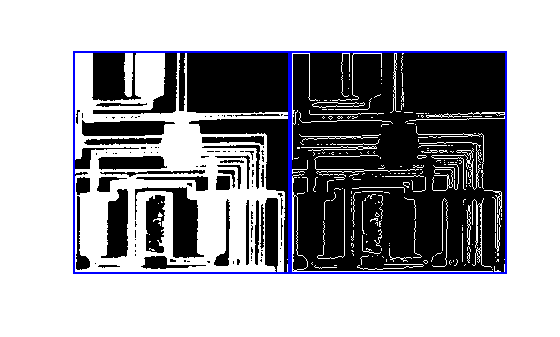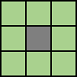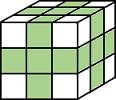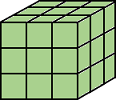# bwperim

Find perimeter of objects in binary image

## Syntax

``BW2 = bwperim(BW)``
``BW2 = bwperim(BW,conn)``
``bwperim(___)``

## Description

example

````BW2 = bwperim(BW)` returns a binary image that contains only the perimeter pixels of objects in the input image `BW`. A pixel is part of the perimeter if it is nonzero and it is connected to at least one zero-valued pixel. ```
````BW2 = bwperim(BW,conn)` also specifies the pixel connectivity, `conn`.```
````bwperim(___)` without output arguments displays the binary image of the perimeter in a new figure window. To use this syntax, `BW` must be a 2-D binary image.```

## Examples

collapse all

Read binary image into workspace.

`BW = imread('circles.png');`

Calculate the perimeters of objects in the image.

`BW2 = bwperim(BW,8);`

Display the original image and the perimeters side-by-side.

`imshowpair(BW,BW2,'montage')`This example shows how to find the perimeter pixels in a binary image using the `bwperim` function.

Read a binary image into the workspace.

`BW1 = imread('circbw.tif');`

Find the perimeters of objects in the image.

`BW2 = bwperim(BW1);`

Display the original image and the image showing perimeters side-by-side.

`montage({BW1,BW2},'BackgroundColor','blue','BorderSize',5)`## Input Arguments

collapse all

Input binary image, specified as a numeric or logical array of any dimension. For numeric input, any nonzero pixels are considered to be `1` (`true`).

Data Types: `single` | `double` | `int8` | `int16` | `int32` | `int64` | `uint8` | `uint16` | `uint32` | `uint64` | `logical`

Pixel connectivity, specified as one of the values in this table. The default connectivity is `4` for 2-D images, and `6` for 3-D images.

Value

Meaning

Two-Dimensional Connectivities

`4`

Pixels are connected if their edges touch. Two adjoining pixels are part of the same object if they are both on and are connected along the horizontal or vertical direction.Current pixel is shown in gray.

`8`

Pixels are connected if their edges or corners touch. Two adjoining pixels are part of the same object if they are both on and are connected along the horizontal, vertical, or diagonal direction.Current pixel is shown in gray.

Three-Dimensional Connectivities

`6`

Pixels are connected if their faces touch. Two adjoining pixels are part of the same object if they are both on and are connected in:

• One of these directions: in, out, left, right, up, and downCurrent pixel is center of cube.

`18`

Pixels are connected if their faces or edges touch. Two adjoining pixels are part of the same object if they are both on and are connected in:

• One of these directions: in, out, left, right, up, and down

• A combination of two directions, such as right-down or in-upCurrent pixel is center of cube.

`26`

Pixels are connected if their faces, edges, or corners touch. Two adjoining pixels are part of the same object if they are both on and are connected in:

• One of these directions: in, out, left, right, up, and down

• A combination of two directions, such as right-down or in-up

• A combination of three directions, such as in-right-up or in-left-downCurrent pixel is center of cube.

For higher dimensions, `bwperim` uses the default value `conndef(ndims(BW),"minimal")`.

Connectivity can also be defined in a more general way for any dimension by specifying a 3-by-3-by- ... -by-3 matrix of `0`s and `1`s. The `1`-valued elements define neighborhood locations relative to the center element of `conn`. Note that `conn` must be symmetric about its center element. See Specifying Custom Connectivities for more information.

Data Types: `double` | `logical`

## Output Arguments

collapse all

Output image containing only perimeter pixels of objects, returned as a logical array.

Data Types: `logical`

## Version History

Introduced before R2006a